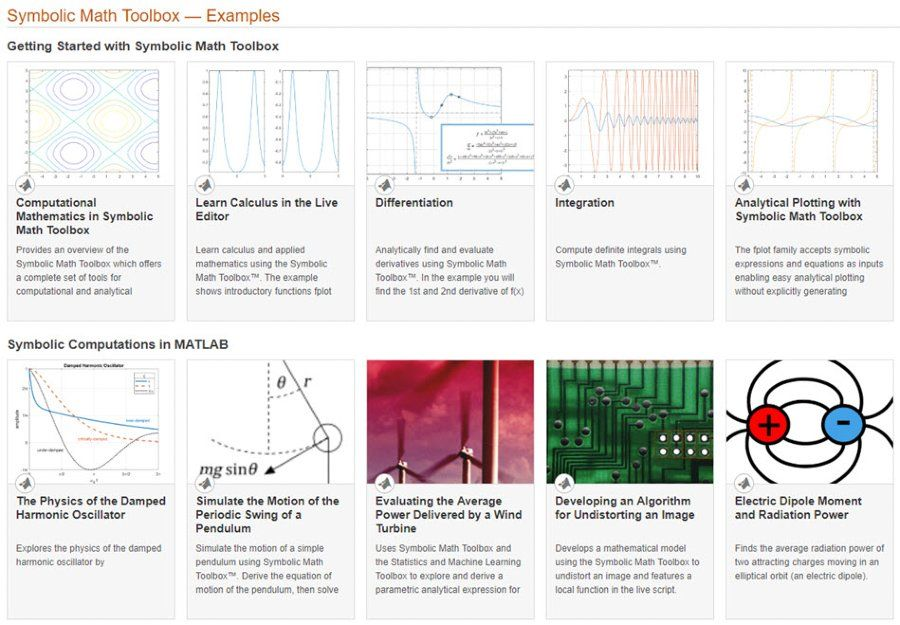## Symbolic math in MATLAB

Symbolic Math Toolbox™ uses the MuPAD® language as part of its underlying computational engine. The language has an extensive set of functions that are optimized for creating and operating on symbolic math expressions.

You can use MATLAB Live Editor to perform, document, and share symbolic math calculations.

### Getting Started with MATLAB Live Editor

MATLAB Live Editor is an interactive way to create, edit, and run MATLAB® code.

In a single environment, your symbolic math computations are displayed in mathematical typeset, along with formatted text, multilingual text, equations, images, and hyperlinks.

You can share live scripts with others to accelerate exploratory analysis, create interactive narratives, and teach with interactive documents.Examples for getting started with Symbolic Math Toolbox and performing symbolic computations in MATLAB.

### Converting MuPAD Notebooks to MATLAB Live Scripts

MATLAB Live Editor is the recommended environment for performing, documenting, and sharing symbolic math computations. MuPAD Notebook functionality is no longer being updated and has been removed in R2020a.

You can use the MATLAB function `convertMuPADNotebook` to convert your MuPAD notebooks to MATLAB live scripts. You can also open a MuPAD notebook as a live script by right clicking and selecting Open As Live Script and save this live script for later use. For further assistance converting your MuPAD notebooks, contact MathWorks SupportConverting a MuPAD Notebook (top, left) to a live script (top, right) using the `convertMuPADNotebook` function (bottom).

The MuPAD notebook has been removed in R2020a. To convert your MuPAD notebook to a MATLAB live script, use the `convertMuPADNotebook` function.